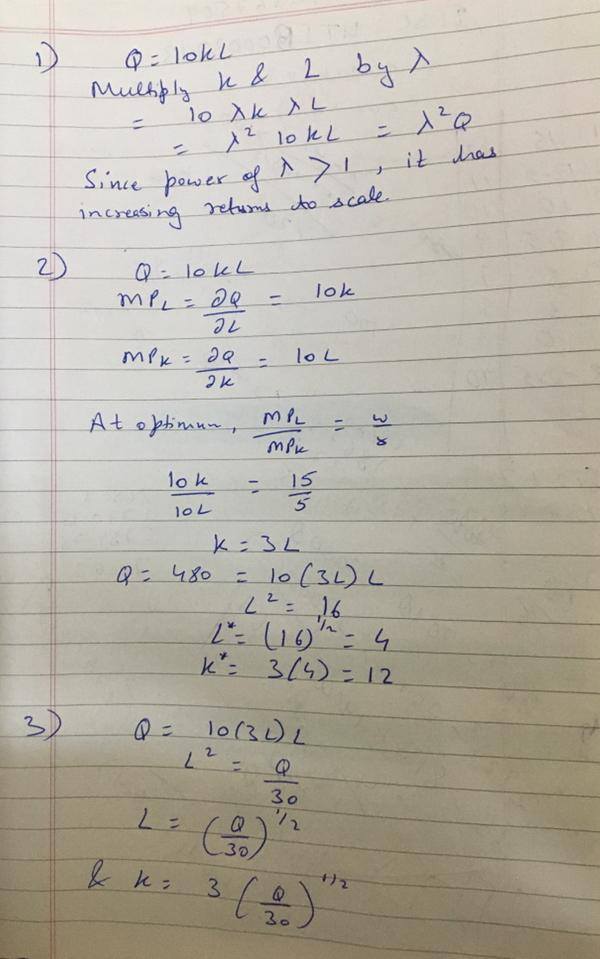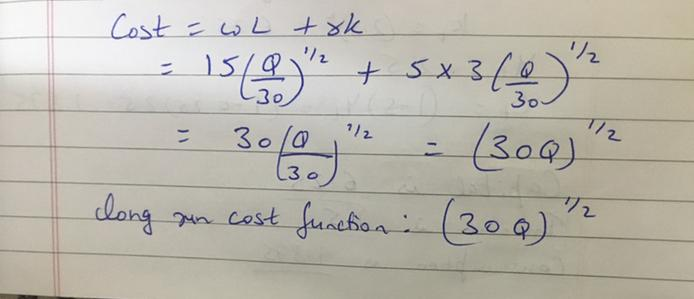In: Economics

# There is a firm who manufacturers and uses capital (K) and labor (L) to product output...

There is a firm who manufacturers and uses capital (K) and labor (L) to product output Q such that Q=10KL. The unit price for K and L are w = $15 and r =$5, respectively.

1).Does the firm’s production exhibit decreasing, constant, or increasing returns to scale?

2)What is the optimal input bundle (K*, L*) to produce 480 unit of output?

3)Derive the long run cost function.

## Solutions

##### Expert Solution## Related Solutions

##### A firm produces output using capital (K) and labor (L). Capital and labor are perfect complements...
A firm produces output using capital (K) and labor (L). Capital and labor are perfect complements and 1 unit of capital is used with 2 units of labor to produce 1 unit of output. Draw an example of an isoquant. If wages and rent are $2 and$3, respectively, what is the Average Total Cost? A firm has a production function given by Q=4KL where K, L and Q denote capital, labor, and output, respectively. The firm wants to produce...
##### Suppose your firm uses 2 inputs to produce its output: K (capital) and L (labor). the...
Suppose your firm uses 2 inputs to produce its output: K (capital) and L (labor). the production function is q = 50K^(1/2)L^(1/2). prices of capital and labor are given as r = 2 and w = 8 a) does the production function display increasing, constant, or decreasing returns to scale? how do you know and what does this mean? b) draw the isoquants for your firms production function using L for the x axis and K for y. how are...
##### A firm discovers that when it uses K units of capital and L units of labor...
A firm discovers that when it uses K units of capital and L units of labor it is able to       produce q=4K^1/4 L^3/4 units of output. Continue to assume that capital and labor can be hired at $40 per unit for labor and$10 for capital. In the long run if the firm produces 600 units of output, how much labor and capital will be used and what is the LR Total cost of production?
##### A firm discovers that when it uses K units of capital and L units of labor...
A firm discovers that when it uses K units of capital and L units of labor it is able to       produce q=4K^1/4 L^3/4 units of output. a) Calculate the MPL, MPK and MRTS b) Does the production function (q=4K^1/4 L^3/4) exhibit constant, increasing or decreasing returns to scale and why? c) Suppose that capital costs $10 per unit and labor can each be hired at$40 per unit and the firm uses 225 units of capital in the short run....
##### Imagine a firm that only uses capital (K) and labor (L). Use an isocost / isoquant...
Imagine a firm that only uses capital (K) and labor (L). Use an isocost / isoquant diagram to illustrate the firm’s equilibrium input mix for given prices of capital and labor and a given rate of output. Now illustrate what happens if the price of labor falls, and the firm wants to produce the same rate of output. What happens to the cost of production? Compare the relative marginal products of labor and capital (the MRTS) at the two equilibria.
##### Suppose output, Q, is produced by labor, L, and capital, K, according to the following function:...
Suppose output, Q, is produced by labor, L, and capital, K, according to the following function: Q = K ½ L½.. Suppose the firm sells each unit of output in a competitive market for a price P = $100. Suppose the firm hires each unit of labor in a competitive market for a wage W =$25. Suppose the firm has to make do for now with a stock of capital K = 49; moreover, suppose each unit of capital...
##### Consider an economy that uses two factors of production, capital (K) and labor (L), to produce...
Consider an economy that uses two factors of production, capital (K) and labor (L), to produce two goods, good X and good Y. In the good X sector, the production function is X = 4KX0.5 + 6LX0.5, so that in this sector the marginal productivity of capital is MPKX = 2KX-0.5 and the marginal productivity of labor is MPLX = 3LX-0.5. In the good Y sector, the production function is Y = 2KY0.5 + 4LY0.5, so that in this sector...
##### A firm produces output y using two factors of production (inputs), labour L and capital K....
A firm produces output y using two factors of production (inputs), labour L and capital K. The firm’s production function is ?(?,?)=√?+√?=?12+?12. The wage rate w = 6 and the rental price of capital r = 2 are taken as parameters (fixed) by the firm. a. Show whether this firm’s technology exhibits decreasing, constant, or increasing returns to scale. b. Solve the firm’s long run cost minimization problem (minimize long run costs subject to the output constraint) to derive this...
##### The production function has two input, labor (L) and capital (K). The price for L and...
The production function has two input, labor (L) and capital (K). The price for L and K are respectively W and V. q = L + K a linear production function q = min{aK, bL} which is a Leontief production function 1.Calculate the marginal rate of substitution. 2.Calculate the elasticity of the marginal rate of substitution. 3.Drive the long run cost function that is a function of input prices and quantity produced.
##### Suppose you are in the photocopying business, and are using labor (L) and capital (K), in...
Suppose you are in the photocopying business, and are using labor (L) and capital (K), in the form of copy machines. You can hire workers for $150 a week and lease copy machines for$300 a week. (a) Suppose your current total cost of production is \$3,000. Use the information above to depict the isocost you are currently operating on, carefully labeling the relevant intercepts (put L on the x-axis and K on the y-axis). (b) You are producing 10,000...